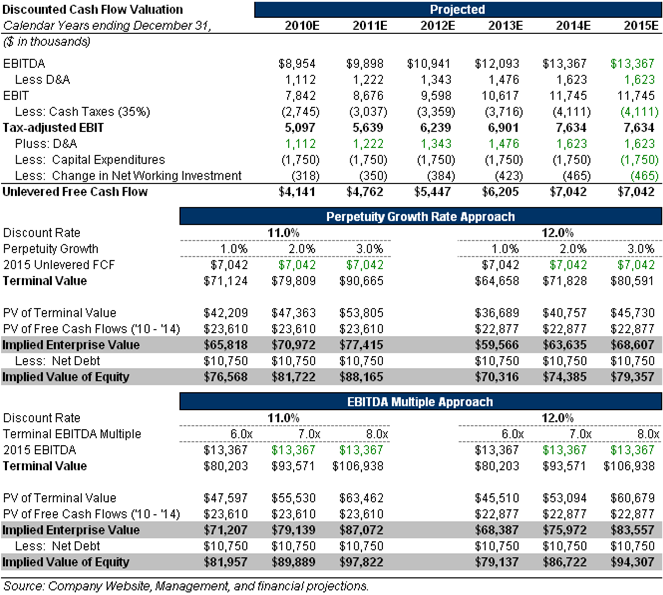# Discounted Cash Flow Modeling

How to model out a discounted cash flow (DCF)  is a must for investment banking interviews: this is the heart and soul of most technical interview questions.  I can guarantee you that you will be asked to walk through a DCF at some point in your banking interviews.

Here’s a quick screen-shot of a real-life DCF Model:What does a DCF Model do?

• A DCF values a company based on the Present Value of its future Cash Flows and the Present Value of its Terminal Value.

What do you usually use for the discount rate?

• WACC is the most common: Weighted Average Cost of Capital.
• Depending on how you setup your DCF you might also use Cost of Equity.

How do you calculate WACC in a DCF model?

• Cost of Equity * (% Equity) + Cost of Debt * (% Debt) * (1 – Tax Rate) + Cost of Preferred * (% Preferred)

How do you calculate Cost of Equity in a DCF model?

• Cost of Equity = Risk-Free Rate + Beta * Equity Risk Premium

What is Beta? What are the formula’s for un-levering and re-levering Beta?

• Beta describes the relation of a stock’s return with that of the financial market as a whole.
• Un-Levered Beta = Levered Beta / (1 + ((1 – Tax Rate) x (Total Debt/Equity)))
• Levered Beta = Un-Levered Beta x (1 + ((1 – Tax Rate) x (Total Debt/Equity)))

Take a deep dive into DCF and other valuation questions in the Investment Banking Technical Guide.

Other interesting articles: